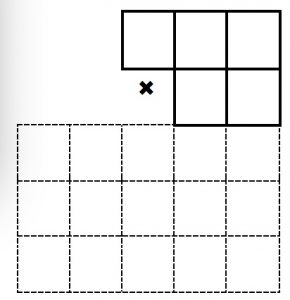Another TARGET game for any number of players. You want to win? So THINK MATHEMATICALLY and ESTIMATE the likely answers. When you multiply H hundreds by T tens what do you want the product of the digits H×T to be? A bit less than 10 perhaps?

You will need a set of 10 cards numbered 0 to 9 or a  0 to 9 spinner.

Spin the spinner and the players must write the digit in one of the top five boxes.

Once a number is written the position cannot be changed.

Repeat four more times.

Then the learners multiply the 3-digit number by the 2-digit number.

The player (or players) with the product closest to 10,000 wins.

Score 1 point for a win.

This is a game of chance but if players think strategically when placing the numbers they can increase their chance of winning.

Alternatively the game can be played by pairs of learners and they will need a spinner for each pair.

Each player draws a multiplication grid like the one shown. Players take turns to spin the spinner. After each spin they each write the number in one of the top 5 boxes of their grid. After 5 spins they multiply their 3-digit number by the 2-digit number and work out the difference between the answer they get and 10,000. The player closest to 10,000 wins that round. The players should check each other’s calculations.

The TARGET 10 THOUSAND Inclusion and Home Learning Guide has the theme ROUNDING AND ESTIMATION. It provides mathematical games suitable for all ages from 5 to 18+.

Click here for the TARGET 10 THOUSAND Inclusion and Home Learning Guide